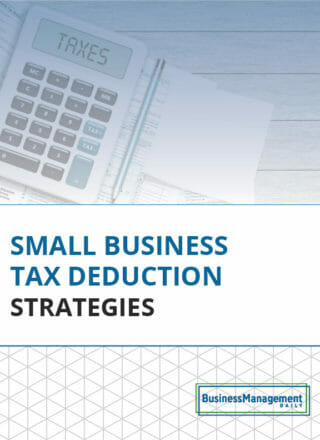# Basing an Excel formula on two conditions

Question: “I need help writing an Excel formula that counts information from two columns. I want the result to be the total number of items that meet two conditions.

Example: Imagine a spreadsheet where Column A records location and Column B records a number. I want to calculate how many entries for Location Such-and-Such also have a number in Column B that is less than 15 . How would I write that formula?” — Valerie

“I came up with the following solution using array formulas. These are special formulas because they act on a range of data called an array.

={SUM((A2:A41=“Midwest”)*(B2:B41<15))}

“The SUM function is used because COUNTIF doesn’t work for this. If you notice, we are multiplying two logical values above. If the first condition in array A is met, then the value is one; otherwise, it is zero. For the second condition in array B, if it is met, the value is one; otherwise, it is zero. So, we raise the SUM by one each time both conditions are met; otherwise, there is no change in the SUM. This turns the SUM command into a counter.

“Array formulas are entered into cells with Control, Shift, Enter, rather than the Enter key alone. Excel will add the curly brackets {} to show that it’s an array formula. If you forget to do that, simply highlight the formula in the formula entry bar and press Control Shift Enter. Don’t type the curly brackets yourself.”

“If I’m understanding your question correctly, the following formula should do what you’re asking.

=IF(B2:B22<15, 1,0)
This is an ‘If Then” statement to determine if column B is < 15 (yes or no, where 1 = YES and 0 = NO).

=IF(C2:C22>0, SUM(C2:C22), “ “)
This is an ‘If Then’ statement to tally the number of cells in column C that are <15 in the selected data range.”